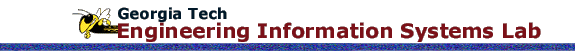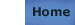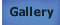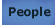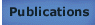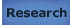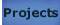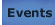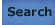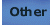# Practical Domain Decomposition Approaches For Parallel Finite Element Analysis (Massively Parallel Computers)

## Reference

Synn, Sang-Youp (1995) Doctoral Thesis, Georgia Institute of Technology, Atlanta.

## Abstract

The objective of this thesis is to explore parallel methodologies for large scale finite element analysis on the massively computers by using the concept of domain decomposition. This research covers the linear static analysis and the nonlinear dynamic analysis. In the linear static analysis, an intelligent strategy is proposed for the global spectrum of engineering computation: initial computation and multiple recomputations. First, simplified formulas are proposed to predict the time complexity in a parallel matrix solver on two machines (BBN,KSR1). Numerical operation counts and data communication costs are considered for those formulas. Based on these formulas, a processor mapping algorithm is proposed to cover the initial computation stage and multiple recomputation stages of substructure analysis, and also compared the performances of the parallel global approach and the parallel substructure approach for several structure models. For nonlinear dynamic analysis, this research tests the generalities of several parallel time integration methods for high-rate nonlinear transient analysis and selects the element level parallelism as the target strategy. Using this parallelism, a large scale general purpose code, DYNA3D is used and modified in several times in order to provide a more detailed parallel implementation strategy. Through several parallel code trials, a basic software design strategy is proposed for massively parallel computers driven by the thread-parallelism.

Manuscript: order via UMI